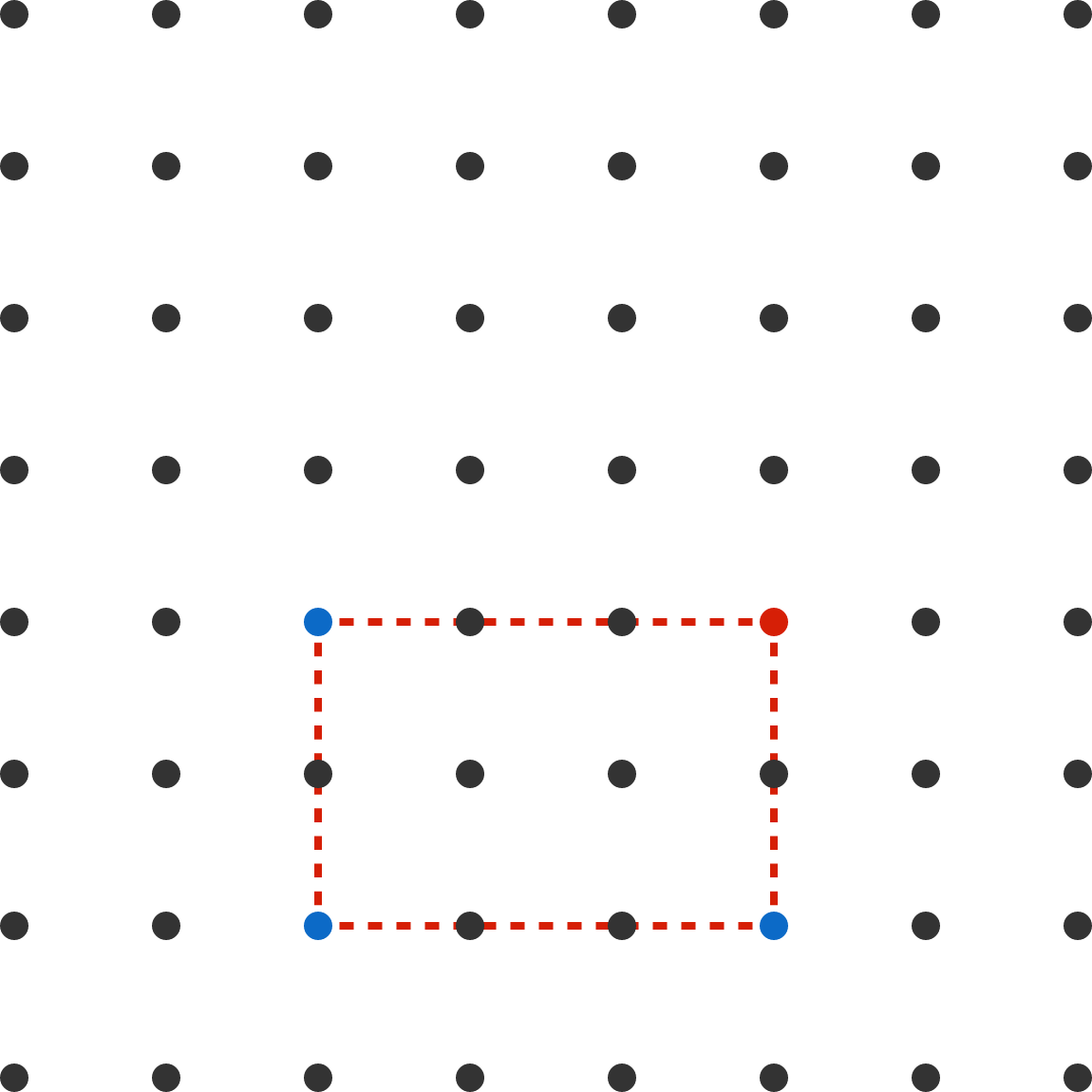Chess board problem

Probability Level 3

In an $8 \times 8$ grid of points, what is the maximum number of points that we can select such that no four selected points are the corners of a rectangle whose sides are parallel to the edges of the grid?If the three blue points are selected, then the red point cannot be selected.

×